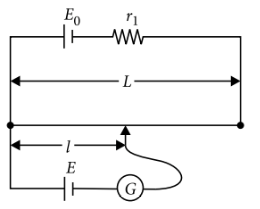potentiometer
Question

# A potentiometer wire of length L and a resistance r are connected in series with a battery of e.m.f.  ${E}_{0}$ and a resistance ${r}_{1}$. An unknown e.m.f. E is balanced at a length $\mathcal{l}$ of the potentiometer wire. The e.m.f. E will be given by

Moderate
Solution

##The current through the potentiometer wire is $I=\frac{{E}_{0}}{\left(r+{r}_{1}\right)}$and the potential difference across the wire is $V=Ir=\frac{{E}_{0}r}{\left(r+{r}_{1}\right)}$The potential gradient along the potentiometer wire is $k=\frac{V}{L}=\frac{{E}_{0}r}{\left(r+{r}_{1}\right)L}$As the unknown e.m.f. E is balanced against length $\mathcal{l}$ of the potentiometer wire,

Get Instant Solutions